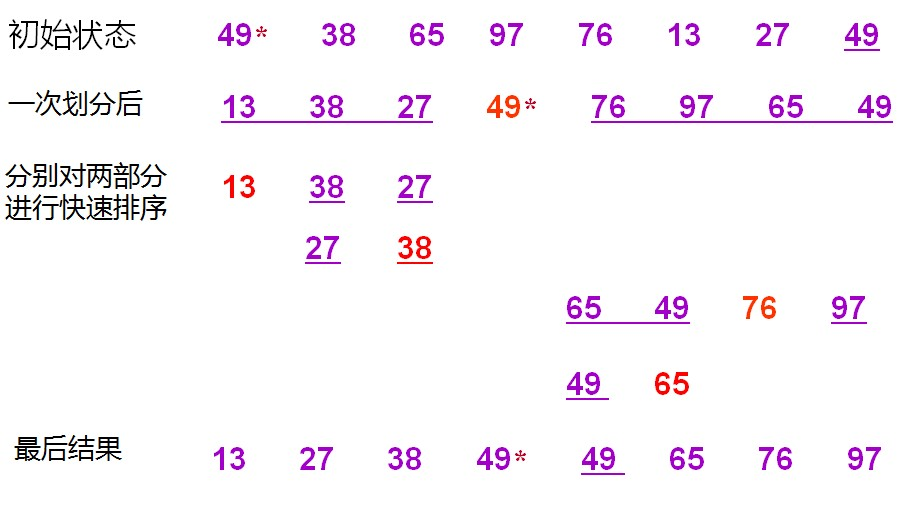﻿ 关于Java排序算法-快速排序（Quick Sort） – 蒋智昊的博客# 关于Java排序算法-快速排序（Quick Sort）```public class SortTest {
public static void main(String[] args) {
int[] arr = { 49, 38, 65, 97, 76, 13, 27, 49 };
display("原始数据 : ", arr);

// 快速排序
quickSort(arr, 0, arr.length - 1);

display("排序结果:", arr);
}

// 交换元素
public static void swap(int[] arr, int a, int b) {
int temp = arr[a];
arr[a] = arr[b];
arr[b] = temp;
}

// 显示
public static void display(String str, int[] arr) {
System.out.println(str);
for (int i = 0; i < arr.length; i++) {
System.out.print(arr[i] + " ");
}
System.out.println();
}

// 快速排序
public static void quickSort(int[] arr, int s, int t) {
// 递归算法，对区间a[s] ～a[t] 进行快速排序
int i = s + 1, j = t;
int temp = 0;
int x = arr[s]; // 第一个为基准元素
while (i <= j) {
// 从左到右
while (i <= j && arr[i] <= x) {
i++;
}

// 从右到左
while (i <= j && arr[j] >= x) {
j--;
}

if (i < j) {
//				temp = arr[i];
//				arr[i] = arr[j];
//				arr[j] = temp;
swap(arr, i, j);
i++;
j--;
}
}

// 交换基准元素
if (s != j) {
arr[s] = arr[j];
arr[j] = x;
}

// 处理左区间
if (s < j - 1) {
quickSort(arr, s, j - 1);
}

// 处理右区间
if (j + 1 < t) {
quickSort(arr, j + 1, t);
}
}

}```

49 38 65 97 76 13 27 49

13 27 38 49 49 65 76 97